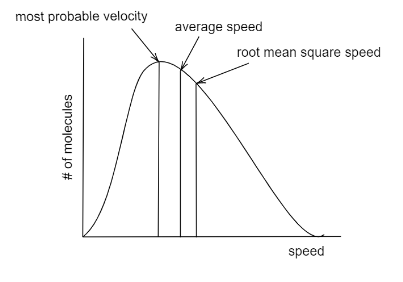Filters
Latest Questions
Mathematics
Chemical kinetics
Write the difference between order and molecularity of reaction.
Chemistry
Chemical kinetics
An organic compound undergoes first-order decomposition. The time taken for its decomposition to 1/8 and 1/10 of its initial concentration at  ${{t}_{\dfrac{1}{8}}}$  and  ${{t}_{\dfrac{1}{10}}}$  respectively. What is the value of  $\dfrac{{{t}_{\dfrac{1}{8}}}}{{{t}_{\dfrac{1}{10}}}}\times 10$ ? (take  ${{\log }_{10}}2=0.3$ )
A. 9
B. 8
C. 10
D. 15
Chemistry
Chemical kinetics
Why does the most-probable speed correspond to the top of the Maxwell-Boltzmann distribution curve, even though the root-mean-square speed is the highest? Why are average and root-mean-square to the right?Chemistry
Chemical kinetics
What equation relates average kinetic energy to temperature in the high-temperature limit?

Chemistry
Chemical kinetics
How does concentration affect ${S_N}2$ reactions?

Chemistry
Chemical kinetics
The temperature coefficient for a certain reaction is $2$ . If the temperature of the reaction is raised by $40$ ${\rm K}$ , what is the approximate factor to which the rate is multiplied?
Chemistry
Chemical kinetics
The order of reaction is decided by
(A) temperature
(B) mechanism of reaction as well as relative concentration of reactants
(C) molecularity
(D) pressure

Chemistry
Chemical kinetics
When a certain amount of ethylene was combusted, $6226$ KJ of the heat was evolved. If heat of combustion of ethylene $1411$ KJ, the volume of oxygen (NTP) that entered into the reaction is
(A) $296.5{\text{ }}ml$
(B) $296.5{\text{ }}litres$
(C) $6226{\text{ }}22.4{\text{ }}litres$
(D) $22.4{\text{ }}litres$

Chemistry
Chemical kinetics
The reaction $A + 2B \to products$ , was found to have the rate law, $rate = k\left[ A \right]{\left[ B \right]^2}$ . Predict by what factor the rate of reaction will increase when the concentration of A and B is doubled?

Chemistry
Chemical kinetics
For the reversible reaction, ${N_{2\left( g \right)}} + 3{H_{2\left( g \right)}} \rightleftharpoons 2N{H_{3\left( g \right)}}$ at ${500^0}C$ , the value of ${K_p}$ is $1.44 \times {10^{ - 5}}$ when partial pressure is measured in the atmosphere. The corresponding value of ${K_c}$ , with concentration in $mol{\left( {litre} \right)^{ - 1}}$
(A) $\dfrac{{1.44 \times {{10}^5}}}{{{{\left( {0.082 \times 500} \right)}^{ - 2}}}}$
(B) $\dfrac{{1.44 \times {{10}^{ - 5}}}}{{{{\left( {8.314 \times 773} \right)}^{ - 2}}}}$
(C) $\dfrac{{1.44 \times {{10}^{ - 5}}}}{{{{\left( {0.082 \times 773} \right)}^2}}}$
(D) $\dfrac{{1.44 \times {{10}^{ - 5}}}}{{{{\left( {0.082 \times 773} \right)}^{ - 2}}}}$
Chemistry
Chemical kinetics
Identify the false statement among the following:
$(a)$ Rate of reaction is directly proportional to activation energy.
$(b)$ Order of reaction can be determined by experimental method only.
$(c)$ Rate law never coincides with the stoichiometry of the reaction.
$(d)$ Order of reaction cort different reactants may be different.
Chemistry
Chemical kinetics
A first order reaction is 50% completed after 30 minutes. This implies that the time required to complete 90% of the reaction is?
Prev
1
2
3
4
5